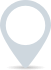# Cost of equity calculator

The cost of equity is a financial metric used to estimate the return required by investors to hold shares of a company’s stock. It represents the minimum rate of return a company must generate to satisfy its shareholders.\$
.00
\$
.00
%

This calculator is intended for illustration purposes only and exact payment terms should be agreed with a lender before taking out a loan.

Cost of equity

-

## How to calculate cost of equity

To calculate the cost of equity, you can use the Capital Asset Pricing Model (CAPM) or the Dividend Discount Model (DDM). I’ll explain both methods:

1. Capital Asset Pricing Model (CAPM): The CAPM calculates the cost of equity based on the risk-free rate of return, the expected market return, and the company’s beta (a measure of the stock’s volatility relative to the overall market).

The formula for calculating the cost of equity using CAPM is:

Cost of Equity = Risk-Free Rate + Beta × (Market Return – Risk-Free Rate)

Here’s how to calculate it:

a. Determine the risk-free rate: Find the current risk-free rate, usually the yield on government bonds, with a similar maturity to the investment horizon.

b. Determine the market return: Estimate the expected return of the overall market, typically using historical market data.

c. Determine the company’s beta: Beta measures the sensitivity of the company’s stock price to market fluctuations. You can find beta values for publicly traded companies from financial websites or databases.

d. Plug in the values into the formula: Substitute the risk-free rate, market return, and company’s beta into the formula to calculate the cost of equity.

1. Dividend Discount Model (DDM): The DDM calculates the cost of equity based on the company’s expected dividends and the expected growth rate of those dividends.

The formula for calculating the cost of equity using DDM is:

Cost of Equity = Dividends per Share / Stock Price + Dividend Growth Rate

Here’s how to calculate it:

a. Determine the expected dividends per share: Estimate the expected dividends the company will pay to its shareholders in the future. This can be based on historical dividends or projected future dividends.

b. Determine the current stock price: Find the current market price per share of the company’s stock.

c. Determine the expected dividend growth rate: Estimate the rate at which the company’s dividends are expected to grow over time.

d. Plug in the values into the formula: Substitute the dividends per share, stock price, and dividend growth rate into the formula to calculate the cost of equity.

Please note that both methods have their limitations and should be used in conjunction with other financial analysis tools when evaluating the cost of equity.

### Clever finance tips and the latest news

#### delivered to your inbox, every week